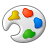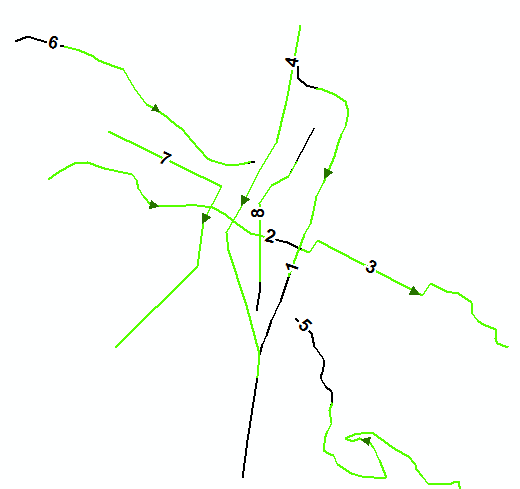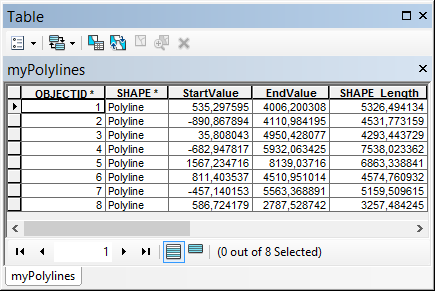# trim line to specified length

2013
5
11-16-2016 07:45 AMby
New Contributor III

Looking for suggestions on trimming polyline features to a specified length starting at a specified distance from the start of the tine. Both values are provided as feature attributes. The resulting features should be perfectly coincident with the original ones, just one end will be removed. Seems like there should be a pre-installed tool for this...am I missing something?

I'll be automating the resulting process (ArcPy?) running on ArcMap 10.1 (soon 10.4) with an Advanced license, in case that opens up any additional possibilities.

Thanks!

Tags (3)
1 Solution

Accepted SolutionsbyMVP Esteemed Contributor

Check the ArcPy Polyline documentation (10.4.x)segmentAlongLine().

5 RepliesbyMVP Esteemed Contributor

Check the ArcPy Polyline documentation (10.4.x)segmentAlongLine().by
New Contributor III

Thanks Joshua. This looks powerful enough to justify waiting for the 10.4 deployment at my workplace.

If anyone else is reading this, I would still be interested in a 10.1 based solution.byEsri Esteemed Contributor

Hi Keith D ,

If I´m correct in 10.1 you have access to the cursors in the data access module and also the polyline::positionAlongLine method. In that case you could do something like:

``````#-------------------------------------------------------------------------------
# Name:        getSegmentAlongLine.py
# Purpose:
#
# Author:      XBAKKER
#
# Created:     16/11/2016
#-------------------------------------------------------------------------------

import arcpy

def main():
fc = r'D:\Xander\GeoNet\GetSegmentAlongLine\gdb\data.gdb\myPolylines'
fld_start = 'StartValue'
fld_end = 'EndValue'
fc_out = r'D:\Xander\GeoNet\GetSegmentAlongLine\gdb\data.gdb\result'

lst_feats = []

flds = ('SHAPE@', fld_start, fld_end)
with arcpy.da.SearchCursor(fc, flds) as curs:
for row in curs:
polyline = row
start_m = row
end_m = row

# create segment along the polyline
segment = getSegmentAlongLine(polyline, start_m, end_m)
lst_feats.append(segment)

arcpy.CopyFeatures_management(lst_feats, fc_out)

def getSegmentAlongLine(polyline, start_m, end_m):
''' get a segment along the line (ArcGIS 10.1)
cut is not available nor is SegmentAlongLine
polyline needs to be projected!

polyline: the input polyline
start_m: start measurement (m)
end_m: end measurement (m)
'''

sr = polyline.spatialReference
length = polyline.length

# correct measurement values
if start_m < 0:
start_m = 0
if end_m > length:
end_m = length
if start_m > end_m:
start_m, end_m = end_m, start_m

# define case
if start_m == end_m:
return None
elif start_m == 0 and end_m == length:
return polyline
else:
# get segment along the line
lst_points = []
len_acum = 0
pnt_prev = None
m = 0
for part in polyline:
for pnt in part:
if pnt_prev == None:
pnt_prev = pnt

# calculate distance between current point and previous one
dist = getDist2Points(pnt_prev, pnt, sr)
m_prev = m
m += dist

if in_range(start_m, m_prev, m):
# start point is between current and previous point
pnt_s = getPointBasedOnRelativePosition(pnt_prev, pnt, sr,
start_m, m_prev, m)
if pnt_s:
lst_points.append(pnt_s.firstPoint)

if in_range_or_equal(m, start_m, end_m):
# point needs to be added to the list
lst_points.append(pnt)

if in_range(end_m, m_prev, m):
# end point is between current and previous point
pnt_e = getPointBasedOnRelativePosition(pnt_prev, pnt, sr,
end_m, m_prev, m)
if pnt_e:
lst_points.append(pnt_e.firstPoint)

pnt_prev = pnt

segment = arcpy.Polyline(arcpy.Array(lst_points), sr)
return segment

def getDist2Points(pnt1, pnt2, sr):
try:
pntg1 = arcpy.PointGeometry(pnt1, sr)
pntg2 = arcpy.PointGeometry(pnt2, sr)
dist = pntg1.distanceTo(pntg2)
return dist
except Exception as e:
print 'ERR getDist2Points', e
return 0

def in_range(value, minimum, maximum):
return value > minimum and value < maximum

def in_range_or_equal(value, minimum, maximum):
return value >= minimum and value <= maximum

def getPointBasedOnRelativePosition(pnt_from, pnt_to, sr, m_pnt, m_from, m_to):
try:
# create line from two points
line = arcpy.Polyline(arcpy.Array([pnt_from, pnt_to]), sr)

# get relative position for m value on line
position = (m_pnt - m_from) / (m_to - m_from)

# return point based on relative position
return line.positionAlongLine(position, True)

except Exception as e:
print 'ERR getPointBasedOnRelativePosition', e
return None

if __name__ == '__main__':
main()
‍‍‍‍‍‍‍‍‍‍‍‍‍‍‍‍‍‍‍‍‍‍‍‍‍‍‍‍‍‍‍‍‍‍‍‍‍‍‍‍‍‍‍‍‍‍‍‍‍‍‍‍‍‍‍‍‍‍‍‍‍‍‍‍‍‍‍‍‍‍‍‍‍‍‍‍‍‍‍‍‍‍‍‍‍‍‍‍‍‍‍‍‍‍‍‍‍‍‍‍‍‍‍‍‍‍‍‍‍‍‍‍‍‍‍‍‍‍‍‍‍‍‍‍‍‍‍‍‍‍‍‍‍‍‍``````

Test results:

• in black the input polylines and indicating with the black labels the OBJECTID of the input features
• in green the resulting lines (indicating the direction too)Based on a featureclass with this attribute table:by
New Contributor III

Thanks for the detailed and thorough response, Xander!byEsri Esteemed Contributor

You're welcome desertsp , however the recommendation remains... it is better to update to a more recent version.